Next: Color Adaptation Up: Appendix Previous: Appendix

## Linear First Order Analysis

In applying our theory of associative decorrelation dynamics to visual cortex to compare with the psychophysical experiments on orientation illusions, the linear first-order approximation is used, which is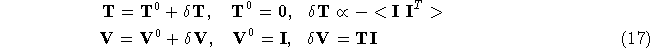where it is assumed that the input correlations are small. It is interesting to notice that the linear first-order approximation leads to anti-Hebbian feedback connections: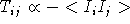which is guarantteed to be stable around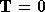.

Let us to illustrate the consequences of this for a simple two neurons case. Suppose the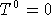, that is the original inputs ensemble is uncorrelated. Now the network is adapted to an input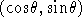, what is the network response to an input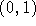now? From equation 17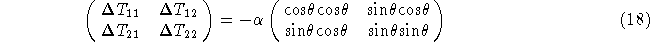and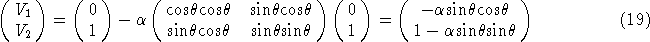So the response after adaptation is actually rotated from the ``vertical'' of input, the angle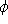rotated is given by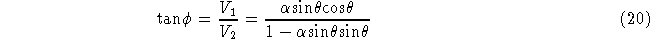Next: Color Adaptation Up: Appendix Previous: Appendix